# Chapter:4-Regression

1. Simple Linear Regression
2. Multiple Linear Regression
3. Polynomial Regression
4. Support Vector for Regression (SVR)
5. Decision Tree Classification
6. Random Forest Classification

# Simple Linear Regression

• h(x_i) represents the predicted response value for ith observation.
• b_0 and b_1 are regression coefficients and represent y-intercept and slope of regression line respectively.
`import numpy as np import matplotlib.pyplot as pltdef estimate_coef(x, y):  # number of observations/points  n = np.size(x)# mean of x and y vector  m_x, m_y = np.mean(x), np.mean(y)# calculating cross-deviation and deviation about x  SS_xy = np.sum(y*x) - n*m_y*m_x  SS_xx = np.sum(x*x) - n*m_x*m_x# calculating regression coefficients  b_1 = SS_xy / SS_xx  b_0 = m_y - b_1*m_xreturn(b_0, b_1)def plot_regression_line(x, y, b):  # plotting the actual points as scatter plot  plt.scatter(x, y, color = "m",    marker = "o", s = 30)# predicted response vector  y_pred = b + b*x# plotting the regression line  plt.plot(x, y_pred, color = "g")# putting labels  plt.xlabel('x')  plt.ylabel('y')# function to show plot  plt.show()def main():  # observations  x = np.array([0, 1, 2, 3, 4, 5, 6, 7, 8, 9])  y = np.array([1, 3, 2, 5, 7, 8, 8, 9, 10, 12])# estimating coefficients  b = estimate_coef(x, y)  print("Estimated coefficients:\nb_0 = {} \   \nb_1 = {}".format(b, b))# plotting regression line  plot_regression_line(x, y, b)if __name__ == "__main__":  main()`
`Estimated coefficients:b_0 = -0.0586206896552b_1 = 1.45747126437`

--

--

--

## More from ABHISHEK KUMAR

Love podcasts or audiobooks? Learn on the go with our new app.

## The 4 Research Techniques to Train Deep Neural Network Models More Efficiently## Quick Glance At Gradient Descent In Machine Learning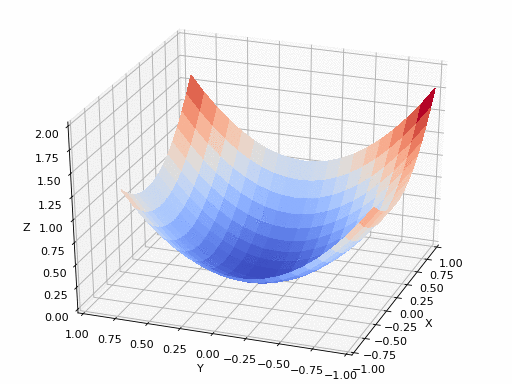## Churn Prediction using Deep Neural Network## Evolution of Neural Machine Translation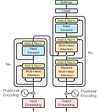## An Introduction to Big Data: Clustering## Tackling the Choice Paralysis for Beginners — Machine Learning by Coursera VS Machine Learning…## M5-Forecasting -Accuracy Top 10% solution## Preparing Deep Learning Interview (I) —  Deep Learning Concept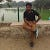## Understand the basics of the Sigmoid function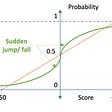## Loss function in machine learning## Random forest: Bagging Example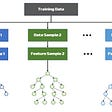## Matching the driver and the rider